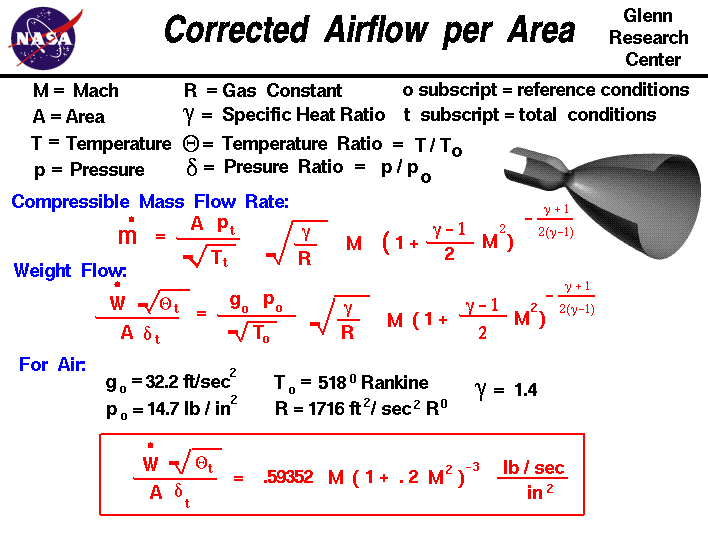+ Text Only Site
+ Non-Flash Version
+ Contact GlennThe conservation of mass is a fundamental concept of physics. Within some problem domain, the amount of mass remains constant; mass is neither created or destroyed. The mass of any object is simply the volume that the object occupies times the density of the object. For a fluid (a liquid or a gas) the density, volume, and shape of the object can all change within the domain with time and mass can move through the domain. The conservation of mass equation tells us that the mass flow rate through a tube is a constant and equal to the product of the density, velocity, and flow area. When we consider the effects of the compressibility of a gas at high speeds we obtain the compressible mass flow equation shown on the slide. mdot = (A * pt/sqrt[Tt]) * sqrt(g/R) * M * [1 + M^2 * (g-1)/2]^-[(g+1)/(g-1)/2] The mass flow rate mdot depends on some properties of the gas (total pressure pt, total temperature Tt, gas constant R, ratio of specific heats g), the area A of the tube, and the Mach number M. With some additional work, we can derive a very useful function which depends only on the Mach number. We begin with the compressible mass flow equation and, using algebra, we divide both sides of the equation by the area, multiply both sides by the square root of the total temperature, and divide both sides by the total pressure. [mdot * sqrt(Tt)]/[A * pt] = sqrt(g/R) * M * [1 + M^2 * (g-1)/2]^-[(g+1)/(g-1)/2] We then multiply both sides by the gravitational constant g0 times a reference pressure p0 divided by the square root of a reference temperature T0. The mass flow rate is changed to a weight flow rate wdot and we assign special symbols; theta to the ratio of the temperature to the reference temperature, and delta to the ratio of the pressure to the reference pressure. The resulting equation is: [wdot * sqrt(thetat)]/[A * deltat] = [g0*p0/sqrt(T0)] * sqrt(g/R) * M * [1 + M^2 * (g-1)/2]^-[(g+1)/(g-1)/2] The right side of the equation now contains the Mach number, the gas constant, the ratio of specific heats, the gravitational constant and the reference pressure and temperature. We pick the reference conditions to be sea level static conditions and the values for the gas properties are given below: g0 = 32.2 ft/sec^2 p0 = 14.7 lb/in^2 T0 = 518 degrees Rankine R = 1716 ft^2/sec^2 degree Rankine g = 1.4 The temperature ratio theta and the pressure ratio delta are just numbers; they have no dimensions. The quantity "w dot times the square root of theta divided by delta" is called the corrected weight flow. If we substitute the numbers given above, we obtain: [wdot * sqrt(thetat)]/[A * deltat] = .59352 * M * [1 + M^2 * (g-1)/2]^-[(g+1)/(g-1)/2] lb/sec/in^2 The corrected weight flow per unit area is just a function of the Mach number. What good is all this? The multiplier (.59352) is computed from reference conditions, so it never changes. The Mach number is a dimensionless property of the flow. We can compute a value for the corrected airflow per unit area at any location in the flow domain. Similarly, if we have a value for the corrected airflow per unit area, we can determine the Mach number (and the velocity) at that location. For some problems, like an ideal nozzle, the area changes but the weight flow stays the same. Using this function we can easily determine the speed at any location. If we add (or subtract) mass, we can recompute the corrected weight flow per area and still determine the velocity. If a shock is present, the total pressure changes. Using the corrected weight flow per unit area function we can determine how the velocity (Mach number) changes by multiplying by the total pressure ratio. If heat is added, or work is done, which changes the total temperature, we can again determine the effects on velocity. This function is extensively used by propulsion engineers to quickly solve duct and nozzle problems. Here is a Java program that solves the corrected airflow equation. This page shows an interactive Java applet to learn how isentropic flows behave by varying the individual flow variables. By default, the program Input Variable is the Mach number of the flow. Since the corrected airflow per area depends only on the Mach number, the program can calculate the value of the airflow per area and display the results on the right side of the output variables. You can also have the program solve for the Mach number that produces a desired value of flow per area. Using the choice button labeled Input Variable, select "Flow per Area Wcor/A". Next to the selection, you then type in a value for Wcor/A. When you hit the red COMPUTE button, the output values change. The corrected airflow is double valued; for the same airflow ratio, there is a subsonic and a supersonic solution. The choice button at the right top selects the solution that is presented. If you are an experienced user of this calculator, you can use a sleek version of the program which loads faster on your computer and does not include these instructions. You can also download your own copy of the program to run off-line by clicking on this button: Activities: Guided Tours Navigation ..Beginner's Guide Home Page+ Inspector General Hotline + Equal Employment Opportunity Data Posted Pursuant to the No Fear Act + Budgets, Strategic Plans and Accountability Reports + Freedom of Information Act + The President's Management Agenda + NASA Privacy Statement, Disclaimer, and Accessibility CertificationEditor: Tom Benson NASA Official: Tom Benson Last Updated: Jun 12 2014 + Contact Glenn